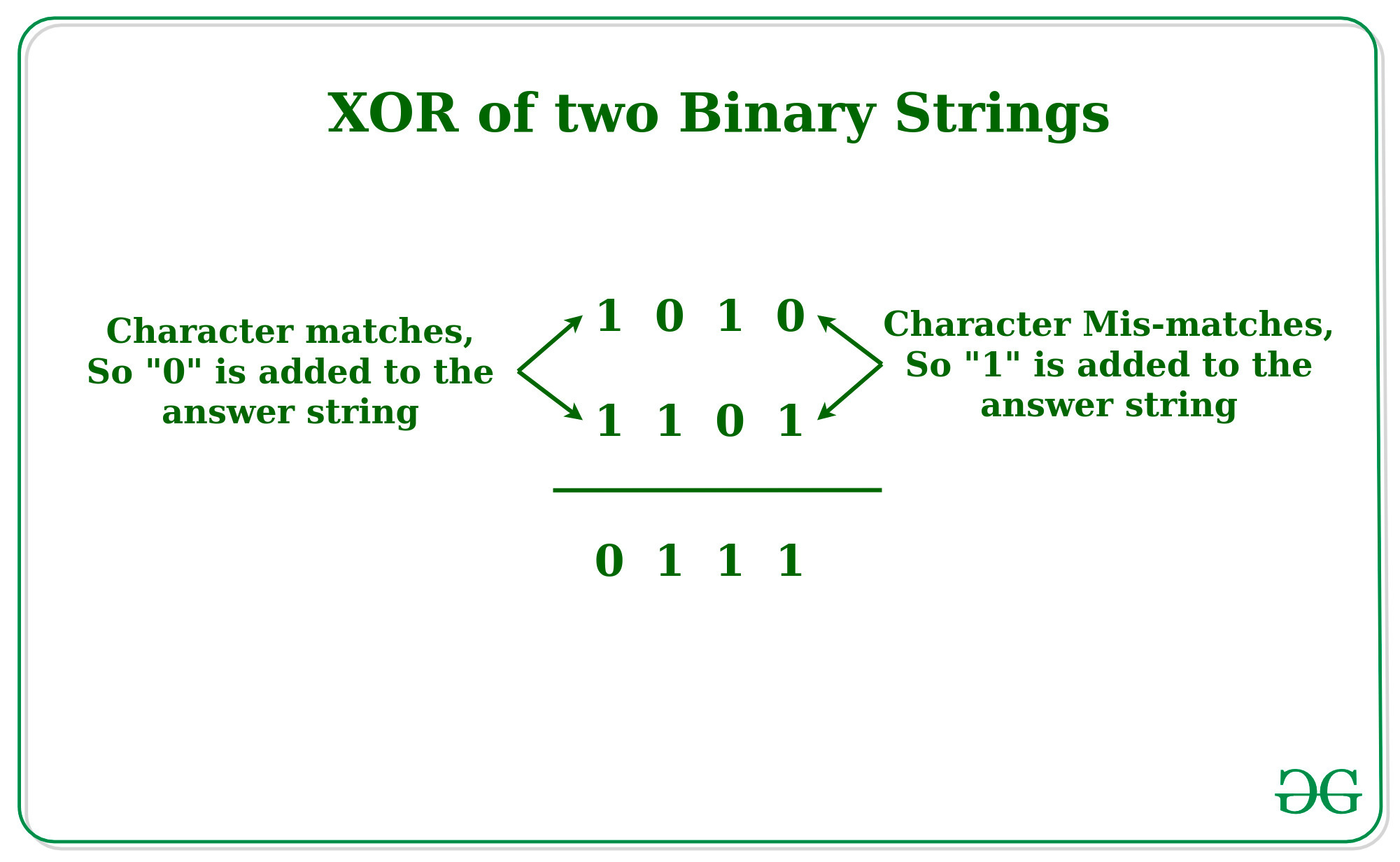# XOR of two Binary Strings

Given two binary strings A and B of equal lengths, the task is to print a string which is the XOR of Binary Strings A and B.

Examples:

Input: A = “0001”, B = “0010”
Output: 0011

Input: A = “1010”, B = “0101”
Output: 1111

## Recommended: Please try your approach on {IDE} first, before moving on to the solution.

Approach: The idea is to iterate over both the string character by character and if the character mismatched then add “1” as the character in the answer string otherwise add “0” to the answer string to generate the XOR string.Below is the implementation of the above approach:

## C++

 `// C++ Implementation to find the  ` `// XOR of the two Binary Strings ` `#include ` `using` `namespace` `std; ` ` `  `// Function to find the  ` `// XOR of the two Binary Strings ` `string xoring(string a, string b, ``int` `n){ ` `string ans = ``""``; ` `     `  `    ``// Loop to iterate over the ` `    ``// Binary Strings ` `    ``for` `(``int` `i = 0; i < n; i++) ` `    ``{ ` `        ``// If the Character matches ` `        ``if` `(a[i] == b[i]) ` `            ``ans += ``"0"``; ` `        ``else` `            ``ans += ``"1"``; ` `    ``} ` `    ``return` `ans; ` `} ` ` `  `// Driver Code ` `int` `main() ` `{ ` `    ``string a = ``"1010"``; ` `    ``string b = ``"1101"``; ` `    ``int` `n = a.length(); ` `    ``string c = xoring(a, b, n); ` `    ``cout << c << endl; ` `} ` ` `  `// This code is contributed by Surendra_Gangwar `

## Java

 `// Java Implementation to find the  ` `// XOR of the two Binary Strings ` `import` `java.io.*; ` ` `  `class` `GFG { ` `    ``// Function to find the  ` `    ``// XOR of the two Binary Strings ` `    ``static` `String  xoring(String a, String b, ``int` `n){ ` `    ``String ans = ``""``; ` `         `  `        ``// Loop to iterate over the ` `        ``// Binary Strings ` `        ``for` `(``int` `i = ``0``; i < n; i++) ` `        ``{ ` `            ``// If the Character matches ` `            ``if` `(a.charAt(i) == b.charAt(i)) ` `                ``ans += ``"0"``; ` `            ``else` `                ``ans += ``"1"``; ` `        ``} ` `        ``return` `ans; ` `    ``} ` `     `  `    ``// Driver Code ` `    ``public` `static` `void` `main (String[] args) ` `    ``{ ` `        ``String a = ``"1010"``; ` `        ``String b = ``"1101"``; ` `        ``int` `n = a.length(); ` `        ``String c = xoring(a, b, n); ` `        ``System.out.println(c); ` `    ``} ` `} ` ` `  `// This code is contributed by shubhamsingh10 `

## Python3

 `# Python Implementation to find the  ` `# XOR of the two Binary Strings ` ` `  `# Function to find the  ` `# XOR of the two Binary Strings ` `def` `xor(a, b, n): ` `    ``ans ``=` `"" ` `     `  `    ``# Loop to iterate over the ` `    ``# Binary Strings ` `    ``for` `i ``in` `range``(n): ` `         `  `        ``# If the Character matches ` `        ``if` `(a[i] ``=``=` `b[i]):  ` `            ``ans ``+``=` `"0"` `        ``else``:  ` `            ``ans ``+``=` `"1"` `    ``return` `ans  ` ` `  `# Driver Code ` `if` `__name__ ``=``=` `"__main__"``: ` `    ``a ``=` `"1010"` `    ``b ``=` `"1101"` `    ``n ``=` `len``(a) ` `    ``c ``=` `xor(a, b, n) ` `    ``print``(c) `

## C#

 `// C# Implementation to find the  ` `// XOR of the two Binary Strings ` `using` `System; ` ` `  `class` `GFG{ ` `    ``// Function to find the  ` `    ``// XOR of the two Binary Strings ` `    ``static` `string` `xoring(``string` `a, ``string` `b, ``int` `n){ ` `    ``string` `ans = ``""``; ` `         `  `        ``// Loop to iterate over the ` `        ``// Binary Strings ` `        ``for` `(``int` `i = 0; i < n; i++) ` `        ``{ ` `            ``// If the Character matches ` `            ``if` `(a[i] == b[i]) ` `                ``ans += ``"0"``; ` `            ``else` `                ``ans += ``"1"``; ` `        ``} ` `        ``return` `ans; ` `    ``} ` `     `  `    ``// Driver Code ` `    ``static` `public` `void` `Main () ` `    ``{ ` `        ``string` `a = ``"1010"``; ` `        ``string` `b = ``"1101"``; ` `        ``int` `n = a.Length; ` `        ``string` `c = xoring(a, b, n); ` `        ``Console.WriteLine(c); ` `    ``} ` `} ` ` `  `// This code is contributed by shubhamsingh10 `

Attention reader! Don’t stop learning now. Get hold of all the important DSA concepts with the DSA Self Paced Course at a student-friendly price and become industry ready.

My Personal Notes arrow_drop_upAmi Munshi is an Asst Professor with MPSTME, Mumbai (NMIMS University) with the Electronics and Telecommunications specialization Focus areas on GfG Application of Python3 libs for Data/Image compression, Encryption, Data Science and Analytics applications

If you like GeeksforGeeks and would like to contribute, you can also write an article using contribute.geeksforgeeks.org or mail your article to contribute@geeksforgeeks.org. See your article appearing on the GeeksforGeeks main page and help other Geeks.

Please Improve this article if you find anything incorrect by clicking on the "Improve Article" button below.